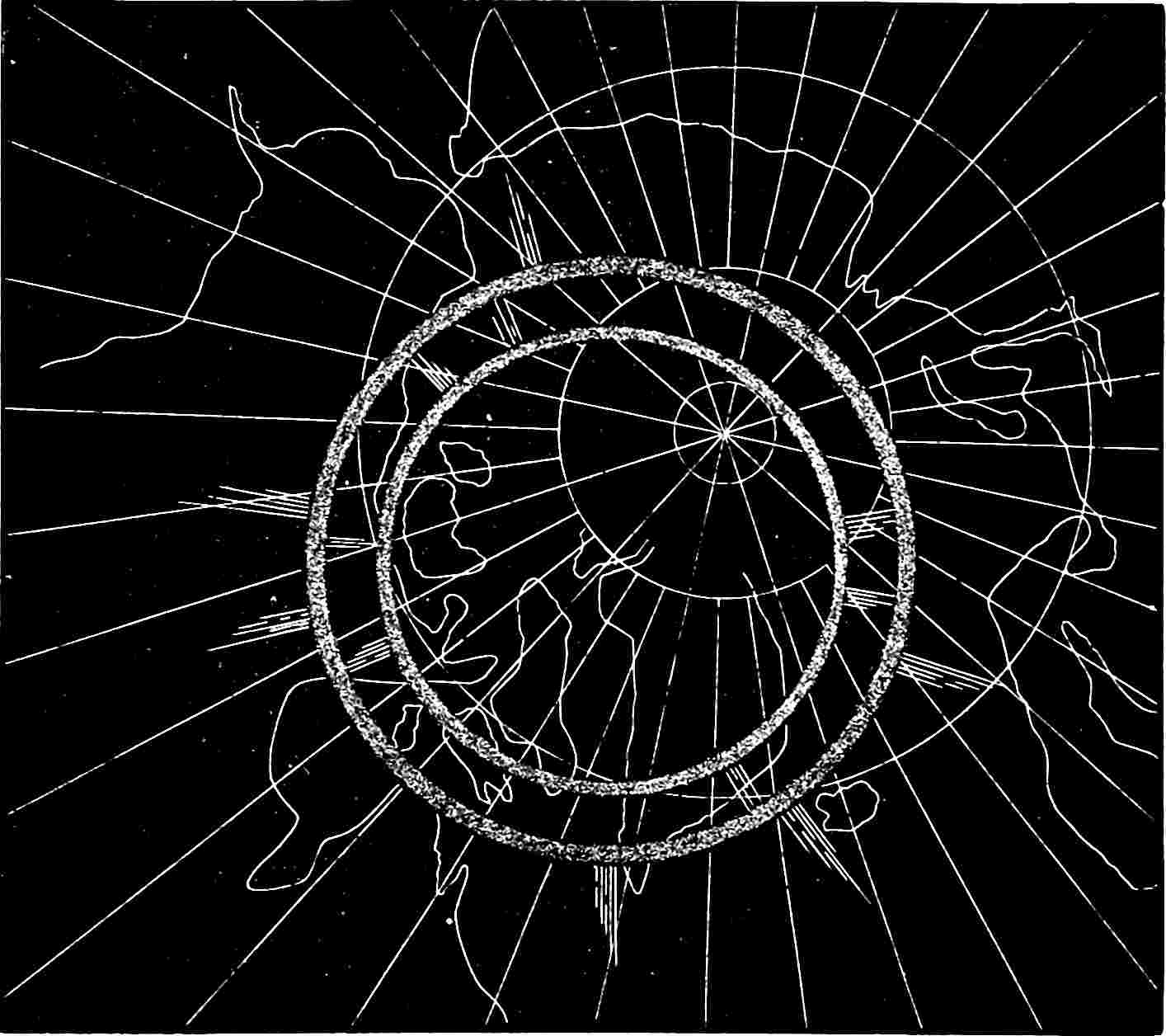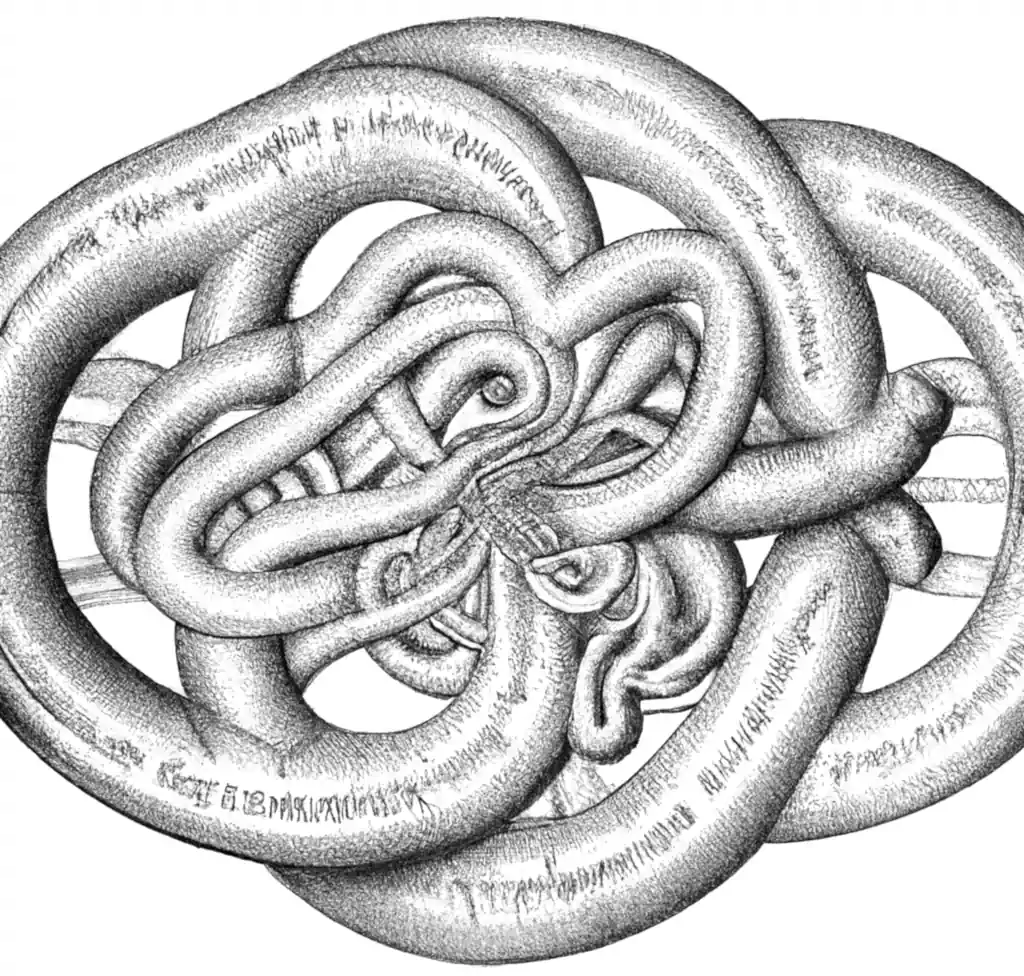## Climbing slower on the tricky bits

A placeholder.The misalignment of the magnetic and geographic poles is not an ideal metaphor for the misalignment of natural and Euclidean gradients (because magnetic field descent leads to the wrong optimum, not the wrong rate) but I can’t be searching online for illustrations all day there is work to do.

Gradient descent with the natural gradient, or a close approximation thereto. Related: Information geometry, which formalises and generalises this. What is that natural gradient then?

The ordinary gradient turns out to be an unnatural direction to follow for variational inference since we are optimizing a distribution, rather than a set of parameters directly. One way to define the gradient is the direction that achieves maximum change subject to a perturbation within a small euclidean ball. To see why the Euclidean distance is an unnatural metric for probability distributions, consider the two Gaussians $$\mathcal{N}(0, 0.1)$$ and $$\mathcal{N}(0, 0.2)$$, compared to $$\mathcal{N} (0, 1000.1)$$ and $$\mathcal{N}(0, 1000.2)$$. The former pair are different and the latter similar, yet in Euclidean distance they are equally far apart in the mean and variance. Using the precision in place of the variance gives the opposite result, yet the distributions are unchanged. There is a fundamental mismatch between the ordinary gradient and the objective function: the gradient is dependent on parameterization whereas the objective function is not.

Fortunately there is a way to solve the disparity: the natural gradient. The natural gradient can be defined as the direction that achieves maximum change in KL divergence. It is well known that paths following the natural gradient are invariant to reparameterization (see e.g., Martens (2020)), and that the natural gradient direction is the ordinary gradient rescaled by the inverse Fisher information matrix .

Martens (2020) says

Natural gradient descent is an optimization method traditionally motivated from the perspective of information geometry, and works well for many applications as an alternative to stochastic gradient descent. In this paper we critically analyze this method and its properties, and show how it can be viewed as a type of approximate 2nd-order optimization method, where the Fisher information matrix can be viewed as an approximation of the Hessian. This perspective turns out to have significant implications for how to design a practical and robust version of the method. Additionally, we make the following contributions to the understanding of natural gradient and 2nd-order methods: a thorough analysis of the convergence speed of stochastic natural gradient descent (and more general stochastic > 2nd-order methods) as applied to convex quadratics, a critical examination of the oft-used “empirical” approximation of the Fisher matrix, and an analysis of the (approximate) parameterization invariance property possessed by natural gradient methods, which we show still holds for certain choices of the curvature matrix other than the Fisher, but notably not the Hessian.

Returning to Salimbeni, Eleftheriadis, and Hensman (2018):

Our fundamental problem is to minimize $$-\mathcal{L}(\xi)$$. All the approaches we consider find a sequence of parameters $$\left\{\boldsymbol{\xi}_{t}\right\}_{t=0}^{T}$$ using the iterative update

$\boldsymbol{\xi}_{t+1}=\boldsymbol{\xi}_{t}-\gamma_{t} \mathrm{P}_{t}^{-1} \mathbf{g}_{t}, \quad \mathbf{g}_{t}=\left.\nabla_{\xi}^{\top} \mathcal{L}\right|_{\xi=\xi_{t}}$ where $$\gamma_{t}$$ denotes the step size and $$\mathrm{P}_{t}^{-1} \mathbf{g}_{t}$$ the direction

Natural gradient descent (NGD). Another way of interpreting the update $$(\sqrt{5})$$ is to use the fact that the direction of steepest descent with respect to a norm $$\|\boldsymbol{\delta}\|_{\mathrm{A}}=\boldsymbol{\delta}^{T} \mathrm{A} \boldsymbol{\delta}$$ is given by $$\mathrm{A}^{-1} \nabla_{\boldsymbol{\xi}}+\mathcal{L}$$. (This can be seen by minimizing $$\frac{1}{\epsilon} \mathcal{L}(\xi+\delta)$$ subject to the constraint that $$\|\boldsymbol{\delta}\|_{\mathrm{A}}=\epsilon$$ and letting $$\epsilon \rightarrow 0$$.) Identifying $$\mathrm{P}$$ with $$\mathrm{A},$$ the update corresponds to the steepest descent with respect to the norm induced by the matrix $$\mathrm{P}$$. Gradient descent (where $$\mathrm{P}$$ is the identity and the induced metric is Euclidean) can therefore be seen as moving in the direction that maximizes the change in objective with respect to the Euclidean norm of the parameters. The Euclidean norm is an unnatural way to compare two parameter vectors if the parameters correspond to distributions, however. If instead we consider the KL divergence between two distributions and take the small perturbation limit, we obtain $$\mathrm{KL}[q(\mathbf{u} ; \boldsymbol{\xi}), q(\mathbf{u} ; \boldsymbol{\xi}+\boldsymbol{\delta})]=\frac{1}{2} \delta^{\top}\left[\mathbb{E}_{q(\mathbf{u} ; \xi)} \nabla_{\xi}^{2} \log q(\mathbf{u} ; \boldsymbol{\xi})\right] \boldsymbol{\delta}+\mathcal{O}\left(\|\boldsymbol{\delta}\|^{3}\right) .$$ Therefore, in a sufficiently small neighbourhood the KL divergence induces a quadratic norm with curvature given by the expected Hessian of the log density. This matrix is known as the Fisher information $$\mathbf{F}_{\xi}$$.

$\mathbf{F}_{\xi}=-\mathbb{E}_{q(\mathbf{u} ; \xi)} \nabla_{\xi}^{2} \log q(\mathbf{u} ; \boldsymbol{\xi})$ The direction of steepest descent with respect to this norm is called the natural gradient $$\tilde{\nabla}_{\xi} \mathcal{L},$$ given by the gradient scaled by the inverse Fisher information: $$\tilde{\nabla}_{\boldsymbol{\xi}} \mathcal{L}=\left(\nabla_{\boldsymbol{\xi}} \mathcal{L}\right) \mathbf{F}_{\boldsymbol{\xi}}^{-1}$$

Ahhhh.